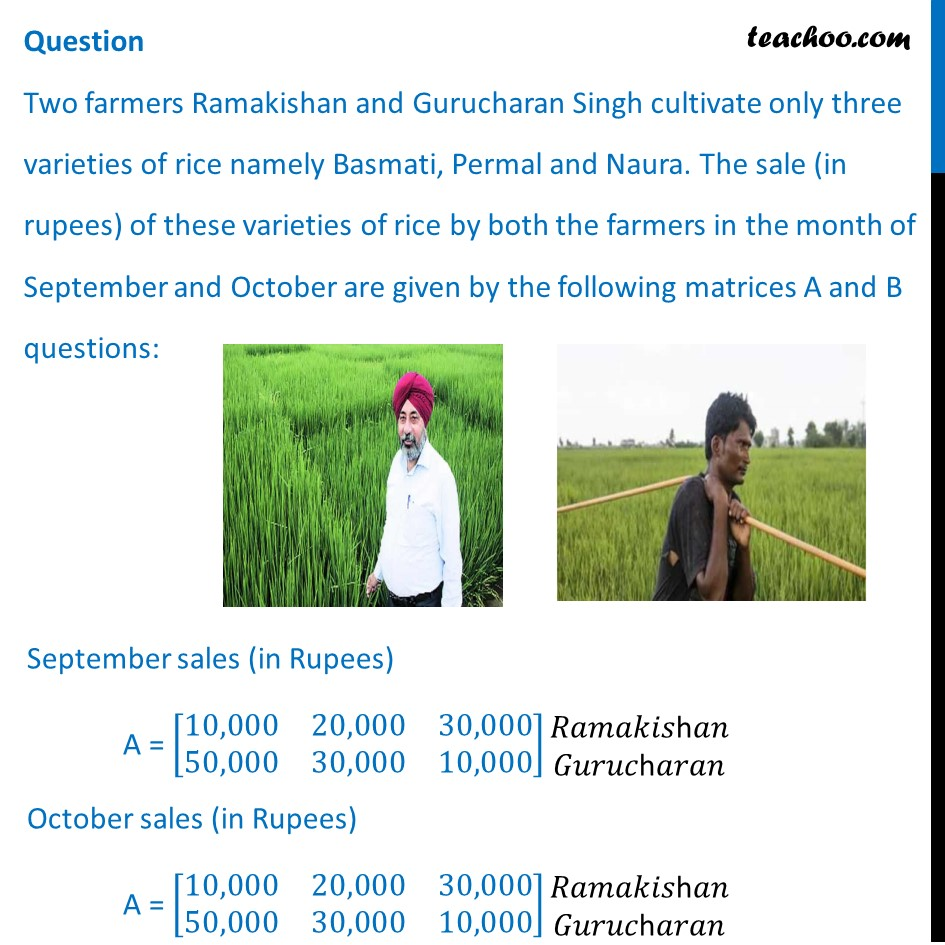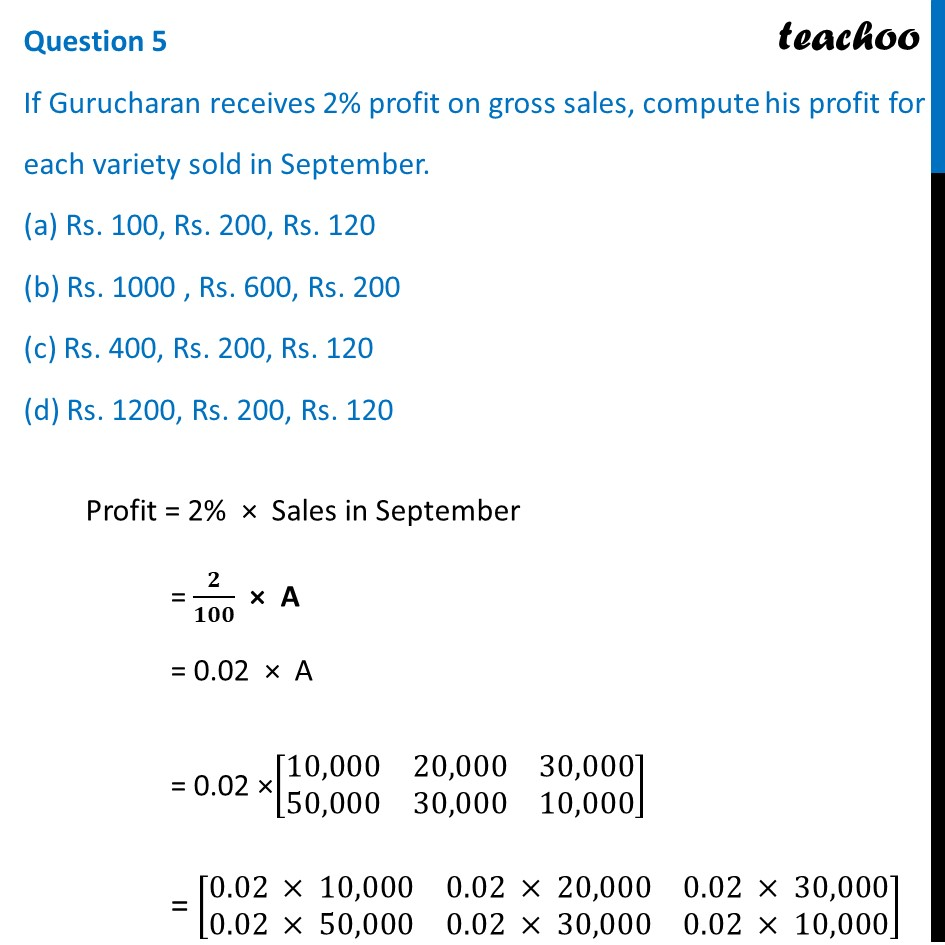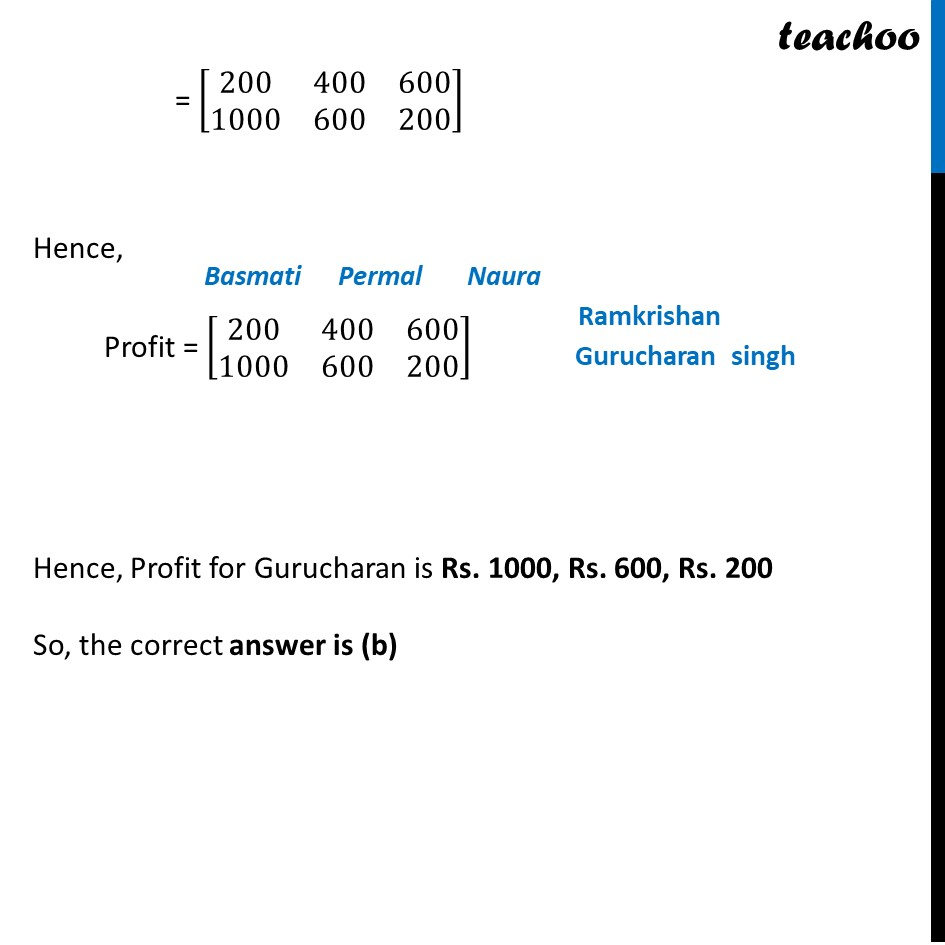Case Based Questions (MCQ)

Chapter 3 Class 12 Matrices
Serial order wise

## Two farmers Ramakishan and Gurucharan Singh cultivate only three varieties of rice namely Basmati, Permal and Naura. The sale (in rupees) of these varieties of rice by both the farmers in the month of September and October are given by the following matrices A and B questions:## October sales (in Rupees)  B =  [ 5,000  10,000  6,000           20,000 10,000 10,000]## (a) Rs. 100, Rs. 200, Rs. 120 (b) Rs. 1000 , Rs. 600, Rs. 200 (c) Rs. 400, Rs. 200, Rs. 120 (d) Rs. 1200, Rs. 200, Rs. 120Note: This questions is inspired from Example 11 - Chapter 3 Class 12

Learn in your speed, with individual attention - Teachoo Maths 1-on-1 Class

### Transcript

Question Two farmers Ramakishan and Gurucharan Singh cultivate only three varieties of rice namely Basmati, Permal and Naura. The sale (in rupees) of these varieties of rice by both the farmers in the month of September and October are given by the following matrices A and B questions: September sales (in Rupees) A = [■8(10,000&20,000&30,000@50,000&30,000&10,000)] ■8("𝑅𝑎𝑚𝑎𝑘𝑖𝑠h𝑎" 𝑛@"𝐺𝑢𝑟𝑢𝑐h𝑎𝑟𝑎" 𝑛) October sales (in Rupees) A = [■8(10,000&20,000&30,000@50,000&30,000&10,000)] ■8("𝑅𝑎𝑚𝑎𝑘𝑖𝑠h𝑎" 𝑛@"𝐺𝑢𝑟𝑢𝑐h𝑎𝑟𝑎" 𝑛) Question 1 The total sales in September and October for each farmer in each variety can be represented as _______. (a) A + B (b) A – B (c) A > B (d) A < B(a) A + B Total sales will be A + B So, the correct answer is (a) Question 2 What is the value of A23? (a) 10000 (b) 20000 (c) 30000 (d) 40000 A = [■8(10,000&20,000&30,000@50,000&30,000&10,000)] So, A23 = 10,000 So, the correct answer is (a) Question 3 The decrease in sales from September to October is given by ______ . (a) A + B (b) A – B (c) A > B (d) A < B Sale in September = A Sale in October = B ∴ Decrease in Sales = A – B So, the correct answer is (b) Question 4 If Ramkishan receives 2% profit on gross sales, compute his profit for each variety sold in October. (a) Rs. 100, Rs. 200 and Rs. 120 (b) Rs. 100, Rs. 200 and Rs. 130 (c) Rs. 100, Rs. 220 and Rs. 120 (d) Rs. 110, Rs. 200 and Rs. 120 Profit = 2% × Sales in October = 𝟐/𝟏𝟎𝟎 × B = 0.02 × B = 0.02 × [■8(5,000&10,000&6,000@20,000&10,000&10,000)] = [■8(0.02 × 5,000&0.02 × 10,000&0.02 × 6,000@0.02 × 20,000&0.02 × 10,000&0.02 × 10,000)] = [■8(100&200&120@400&200&200)] Hence, Profit = [■8(100& 200& 120@400& 200& 200)] Hence, Profit for Ramkrishan is Rs. 100, Rs. 200 and Rs. 120 So, the correct answer is (a) Question 5 If Gurucharan receives 2% profit on gross sales, compute his profit for each variety sold in September. (a) Rs. 100, Rs. 200, Rs. 120 (b) Rs. 1000 , Rs. 600, Rs. 200 (c) Rs. 400, Rs. 200, Rs. 120 (d) Rs. 1200, Rs. 200, Rs. 120 Profit = 2% × Sales in September = 𝟐/𝟏𝟎𝟎 × A = 0.02 × A = 0.02 ×[■8(10,000&20,000&30,000@50,000&30,000&10,000)] = [■8(0.02 × 10,000&0.02 × 20,000&0.02 × 30,000@0.02 × 50,000&0.02 × 30,000&0.02 × 10,000)] = [■8(200&400&600@1000&600&200)] Hence, Profit = [■8(200&400&600@1000&600&200)] Hence, Profit for Gurucharan is Rs. 1000, Rs. 600, Rs. 200 So, the correct answer is (b)